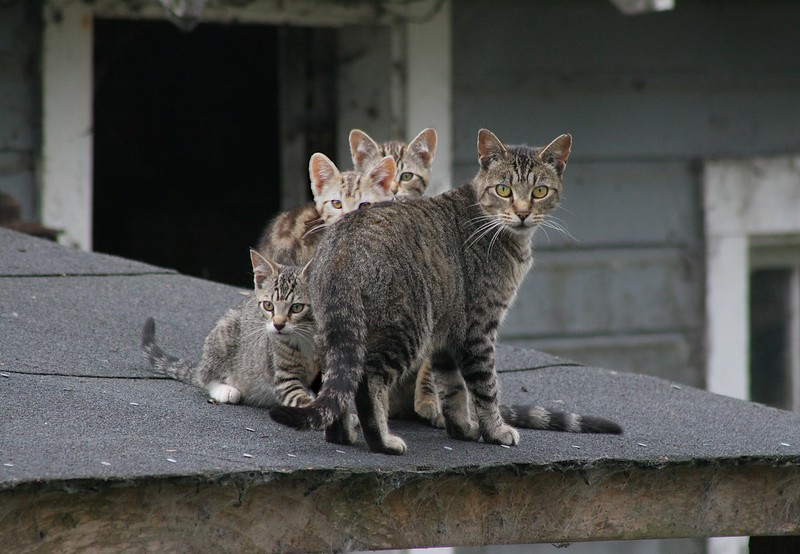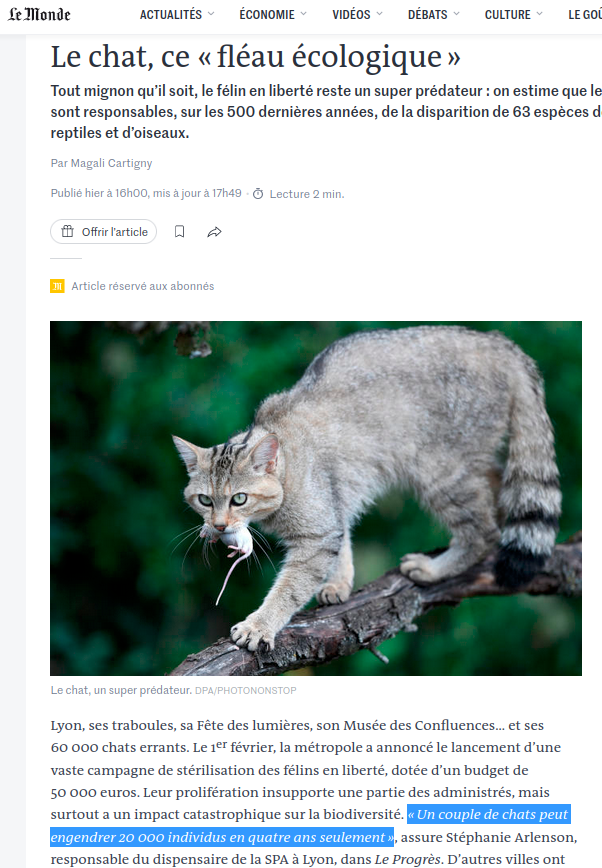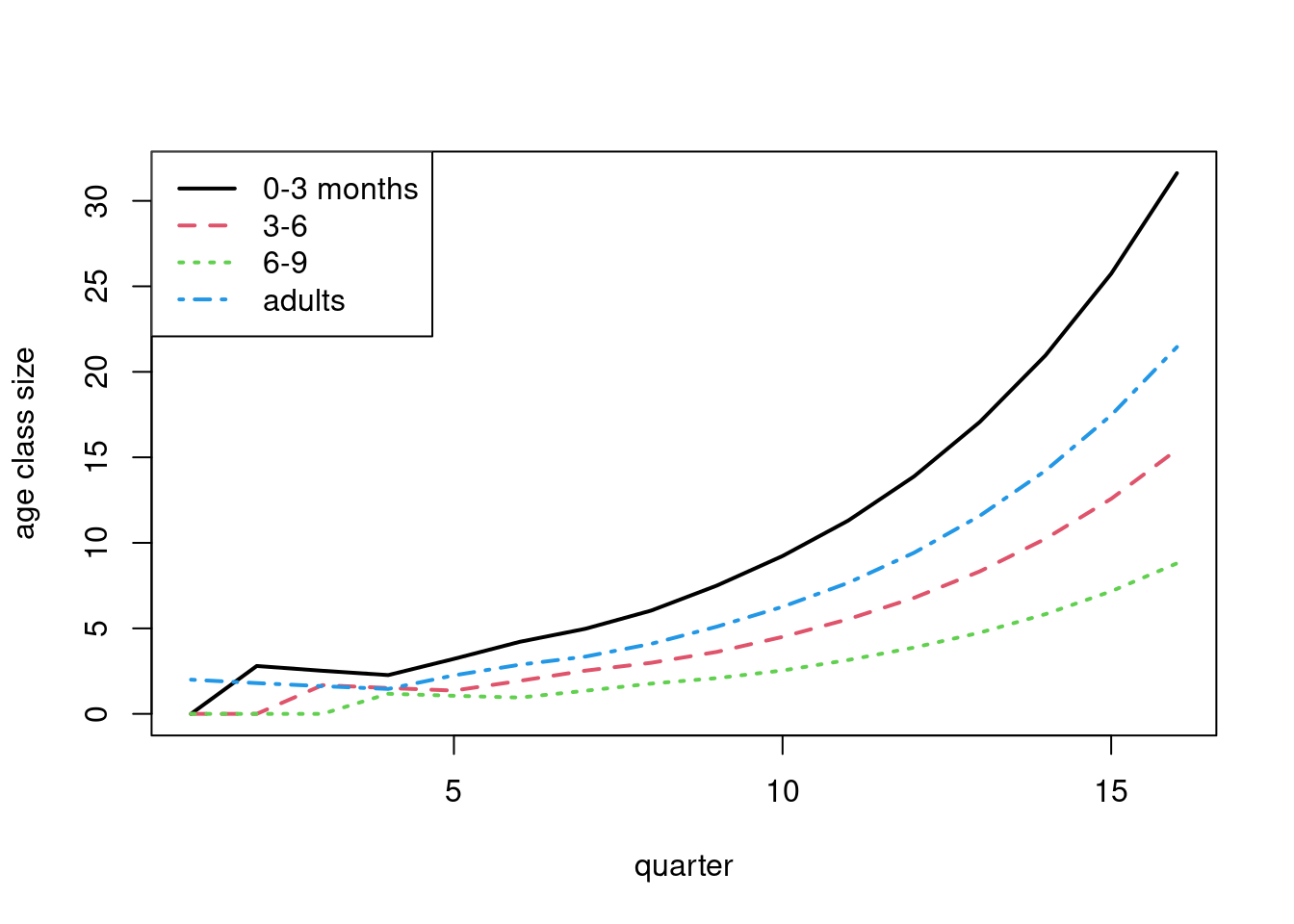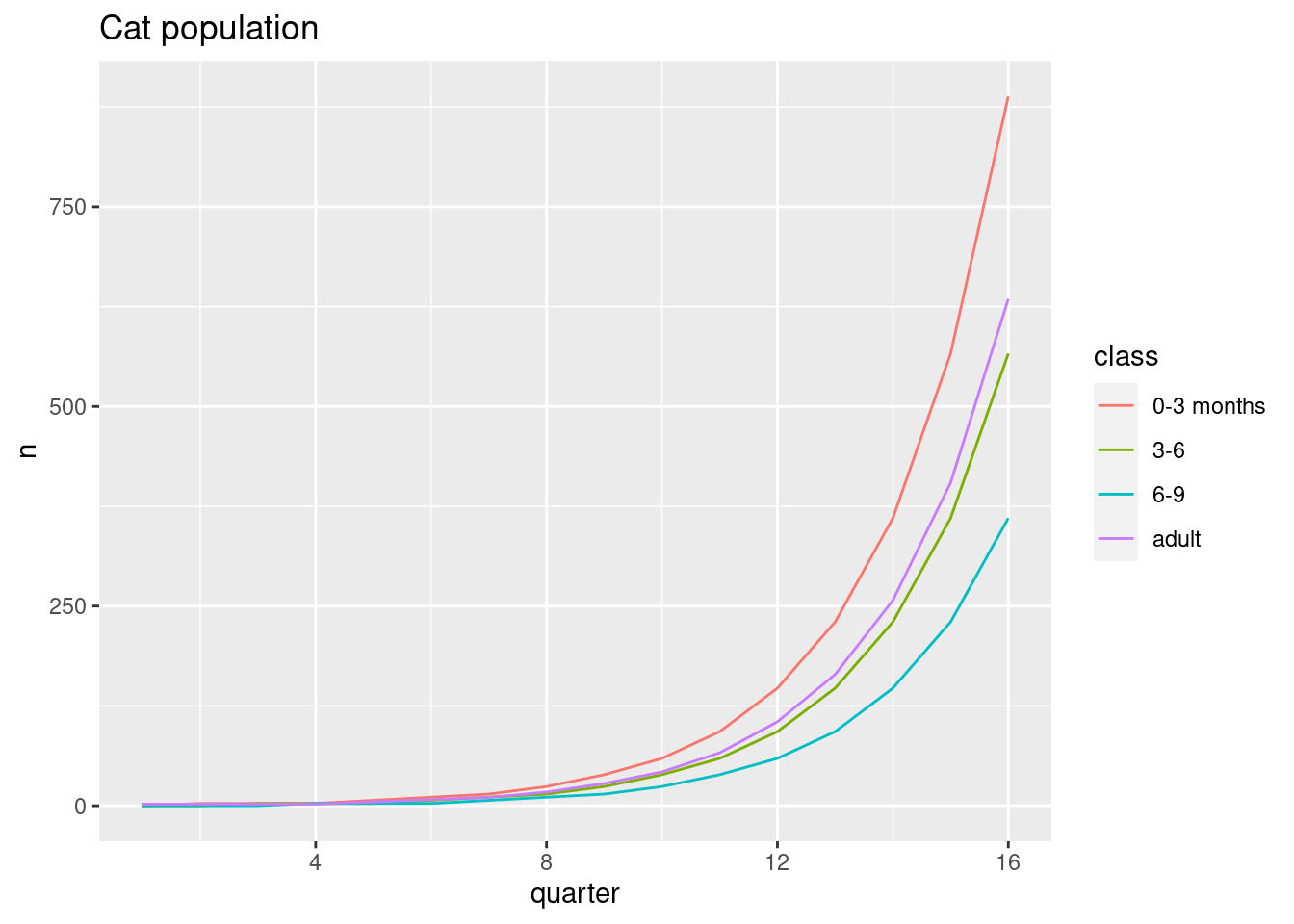# Population growth

Cats, many cats
R
ecology
Author

Michaël

Published

2023-02-10

Modified

2023-09-22Feral Cat Mom and 3 Kittens – CC BY-NC-ND by Chriss Pagani« a pair of cats can produce 20,000 individuals in just four years ».

(translation)

That seems quite high… Let’s check!

Note

Additionally, the article is about feral cats preying on wildlife but we are shown a picture of a wild cat capturing a laboratory mouse!

This figure could come from a back-of-the-envelope calculation, such as litters of 3.5 kitten twice a year for 4 years producing 3.58 ≈ 22,519 kitten. But that’s a very rough and false estimate: at this rate there will be 76 billions cats in Lyon in 10 years! Moreover we ignore the fact that the first generations can still have litters, the less than perfect survival of feral cats, the delay before the first pregnancy, etc.

We can use Leslie matrix to model the destiny of our founding pair. Leslie matrices are used in population ecology to project a structured (by age) population based on transitions between age classes and fertility.

Cat females reach sexual maturity at 6–8 months , can have 2.1 litters each year and have a mean of around 4 kitten by litter or 9.1 kitten by year . So we will use quarters as ages classes. We first use an unrealistic 100 % survival and 100 % fecundity.

## R base version

The first line of the matrix reads 0 kitten produced between 0-3 months, 0 kitten between 3-6 months, and $$9.1 / 2 / 4 = 1.4$$ kitten by capita by quarter for the 6-9 months class and the adults. The 1s on the diagonal are the survivals between age classes and the lower-right 1 the adult survival.

l <- matrix(c(0, 0, 1.4, 1.4,
1, 0,   0,   0,
0, 1,   0,   0,
0, 0,   1,   1),
nrow = 4,
byrow = TRUE)

quarters <- 16

n0 <- matrix(c(0, 0, 0, 2),
ncol = 1)

simul <- function(q) {
n_proj <- matrix(0,
nrow = nrow(l),
ncol = q)
n_proj[, 1] <- n0

for (i in 1:(q - 1)) {
n_proj[, i + 1] <- l %*% n_proj[, i]
}
return(n_proj)
}

res <- simul(quarters)
(final_pop <- round(sum(res[, ncol(res)])))
 2449

With our optimistic parameters, we get a population of around 2450 cats after 16 quarters (4 years). An order of magnitude less than in the article…

With a more realistic matrix, especially for feral cats, with less fertility for the first pregnancy and survival rates totally made-up but not 100 %, we can get quite a more manageable population size:

l <- matrix(c(0,     0,   1, 1.4,
0.6,   0,   0,   0,
0,   0.7,   0,   0,
0,     0, 0.8,  .9),
nrow = 4,
byrow = TRUE)

res <- simul(quarters)
(final_pop_2 <- round(sum(res[, ncol(res)])))
 77
round(res)
     [,1] [,2] [,3] [,4] [,5] [,6] [,7] [,8] [,9] [,10] [,11] [,12] [,13] [,14]
[1,]    0    3    3    2    3    4    5    6    8     9    11    14    17    21
[2,]    0    0    2    2    1    2    3    3    4     5     6     7     8    10
[3,]    0    0    0    1    1    1    1    2    2     3     3     4     5     6
[4,]    2    2    2    1    2    3    3    4    5     6     8     9    12    14
[,15] [,16]
[1,]    26    32
[2,]    13    15
[3,]     7     9
[4,]    17    21
matplot(1:quarters, t(res), type = "l", lty = 1:ncol(l),
xlab = "quarter", ylab = "age class size", lwd = 2)
legend("topleft", legend = c("0-3 months", "3-6", "6-9", "adults"),
lty = 1:ncol(l), col = 1:ncol(l), lwd = 2)So only 77 cats now… I let you play with fertility and survival rates to stabilize the population.

But anyway, cats do have an effect on wildlife, so whatever their population size, we must act to reduce their impact.

## Tidyverse version

library(tidyverse)

l <- matrix(c(0, 0, 1.4, 1.4,
1, 0,   0,   0,
0, 1,   0,   0,
0, 0,   1,   1),
nrow = 4,
byrow = TRUE)

quarters <- 16

n0 <- matrix(c(0, 0, 0, 2),
ncol = 1)

res <- list(l) |>
rep(quarters - 1) |>
accumulate(%*%, .init = n0, .dir = "backward") |>
rev() |>
bind_cols(.name_repair = "unique_quiet")

res |>
summarise(across(last_col(), sum))
# A tibble: 1 × 1
...16
<dbl>
1 2449.
res |>
mutate(class = c("0-3 months", "3-6", "6-9", "adult"), .before = 1) |>
pivot_longer(-1,
names_to = "quarter",
values_to = "n",
names_prefix = "...",
names_transform = as.integer) |>
ggplot(aes(quarter, n)) +
geom_line(aes(color = class)) +
labs(title = "Cat population")